# Overview of Set Theory

• 第1章 数学语言与证明方法
• 第4章 关系
• 第5章 函数

🤔 集合论部分的特点是，所有的数学元素都可以用集合来表示，比如关系是有序对的集合，而函数则是特殊的关系。这于初等数学的理解是不同的。

🤔 对称差类似于异或运算

## 关系

### 有序对和笛卡尔积

$$A \times B = {\left\langle a, b \right\rangle|a\in A \and b \in B}$$

🤔 笛卡尔积的性质显而易见了兄弟们

### 二元关系

1. 集合非空，它的元素都是有序对
2. 集合是空集

🤔 $xRy$可以看成是逻辑值

### 重要关系

#### 全域关系

$$E_A={\langle x,y\rangle|x\in A\land y\in A}=A\times A$$

#### 恒等关系

$$I_A={\langle x,x\rangle|x\in A}$$

### 关系的表示

🤔 有序对的集合列举

#### 关系图

$A$上关系$R$的关系图是有向无权图$G_R=\langle A,R\rangle$，其中 A 为 G 的节点集

### 关系运算

#### 运算定理

• $(F^{-1})^{-1}=F$
• $domF^{-1}=ranF,ranF^{-1}=domF$

• $(F\circ G)\circ H=F\circ(G\circ H)$
• $(F\circ G)^{-1}=G^{-1}\circ F^{-1}$

• $R\circ I_A=I_A\circ R=R$

#### 幂运算

1. $$R^0={\langle x,x\rangle|x\in A}=I_A$$
2. $$R^{n+1}=R^n\circ R$$

🤔 这里可以用于关系的矩阵运算

### 关系的性质

🤔 重难点，这里衍生出了特殊的关系

#### 定义及判别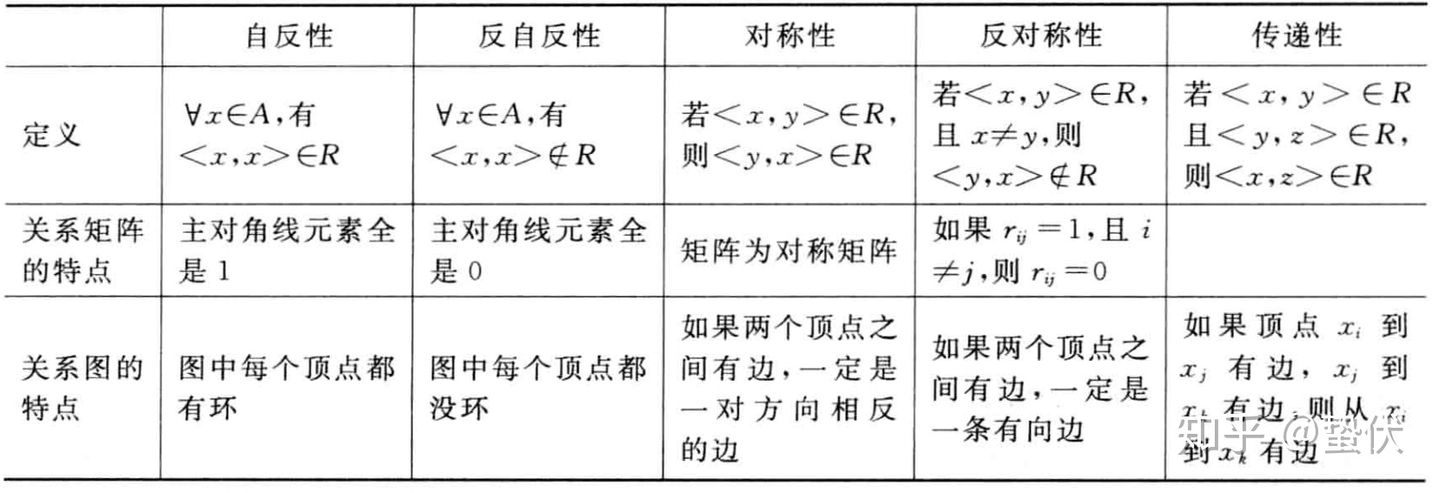#### 自反

• 若$\forall x(x\in A\rightarrow\langle x,x\rangle \in R)$，则称$R$在$A$上自反
• 若$\forall x(x\in A\rightarrow\langle x,x\rangle \notin R)$，则称$R$在$A$上反自反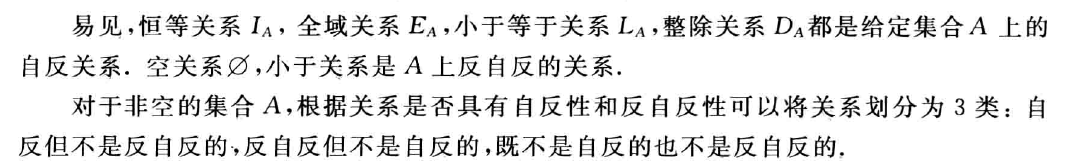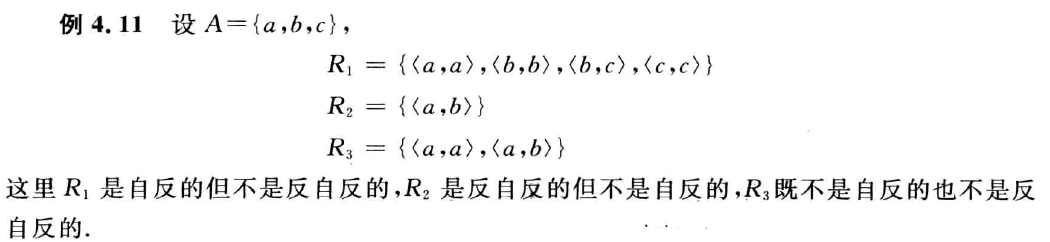#### 对称

• 若 $\forall x\forall y(x,y\in A\ \land\langle x,y\rangle \in R\rightarrow \langle y,x\rangle\in R)$，则称$R$在$A$上对称
• 若 $\forall x\forall y(x,y\in A\ \land\langle x,y\rangle \in R\land \langle y,x\rangle\in R\rightarrow x=y)$，则称$R$在$A$上反对称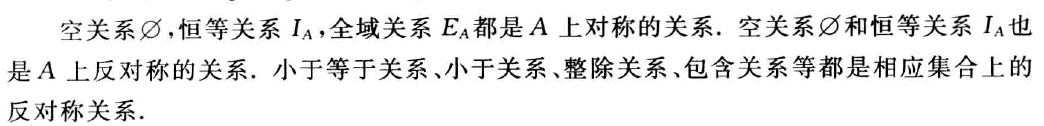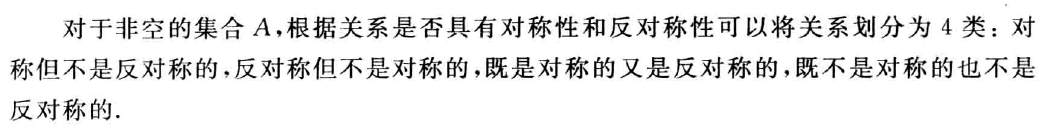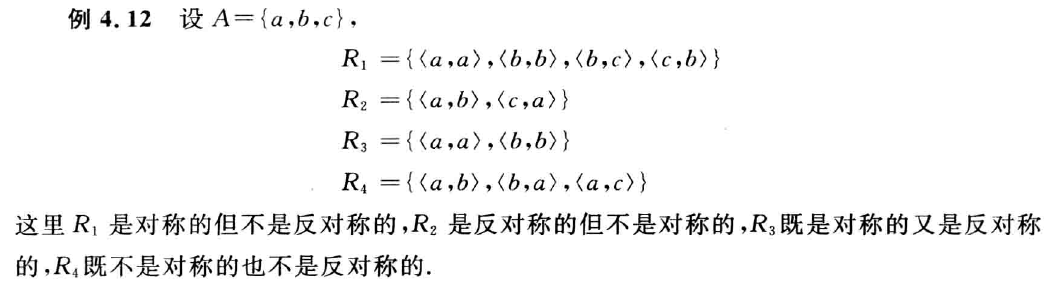#### 传递

$$\forall x\forall y\forall z(x,y,z\in A\land \langle x,y\rangle\in R\land \langle y,z\rangle\in R\rightarrow \langle x,z\rangle\in R)$$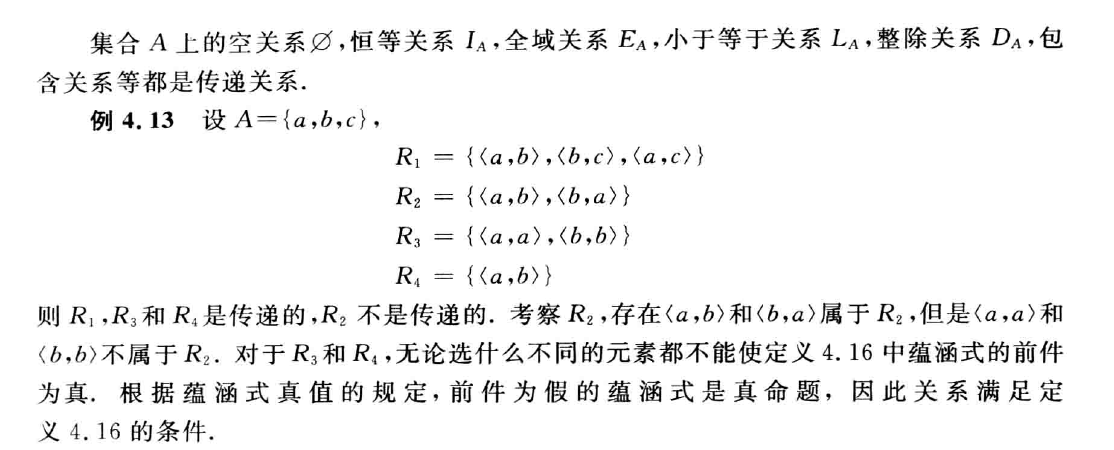#### 判别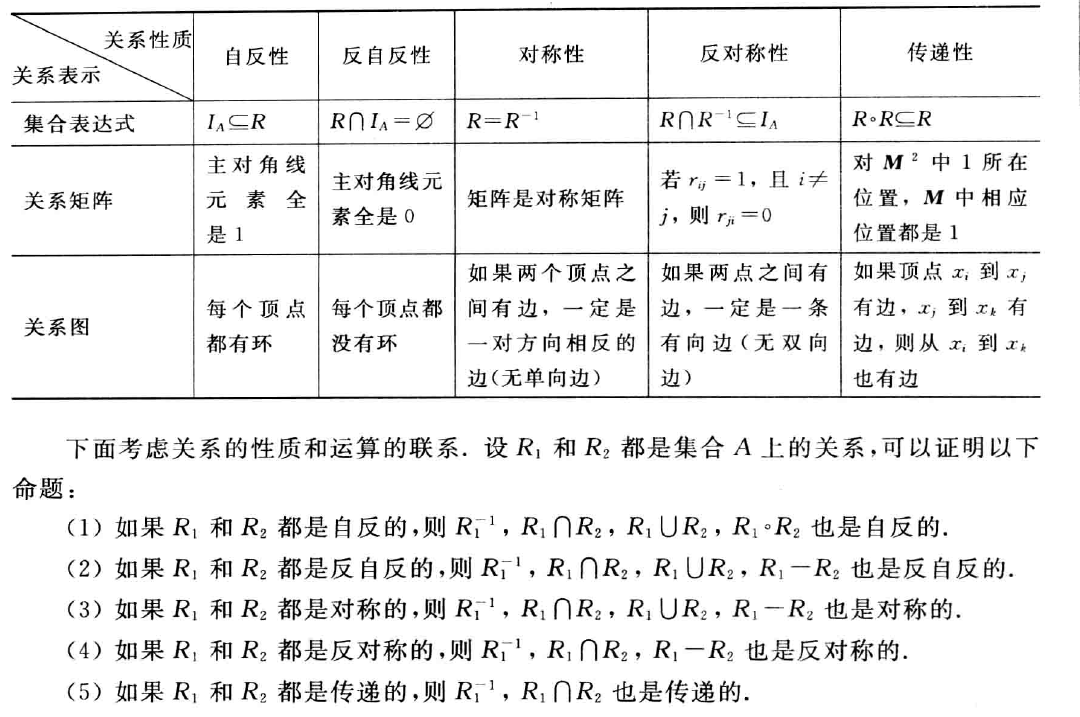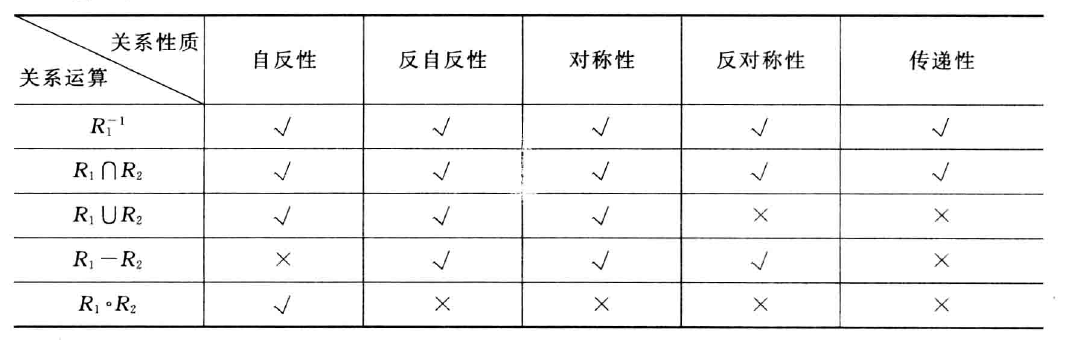### 关系的闭包

#### 定义

• $R’$是自反的（对称的或传递的）
• $R\subseteq R’$
• 对$A$上任何包含$R$的自反（对称或传递）关系$R’’$有$R’\subseteq R’’$

#### 定理

• $r(R)=R\cup R^0$
• $s(R)=R\cup R^{-1}$
• $t(R)=R\cup R^2\cup R^3\cup\ldots$

• $M_r=M+E$
• $M_S=M+M’$
• $M_t=M+M^2+M^3+\ldots$

• $G_r$：在图$G$中每一个缺少环的结点都加一个环
• $G_s$：将$G$中的单向边变成双向边
• $G_t$：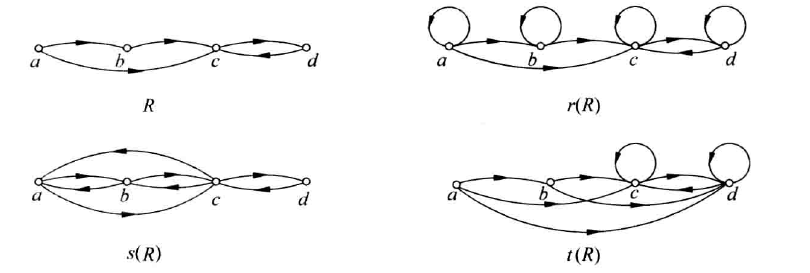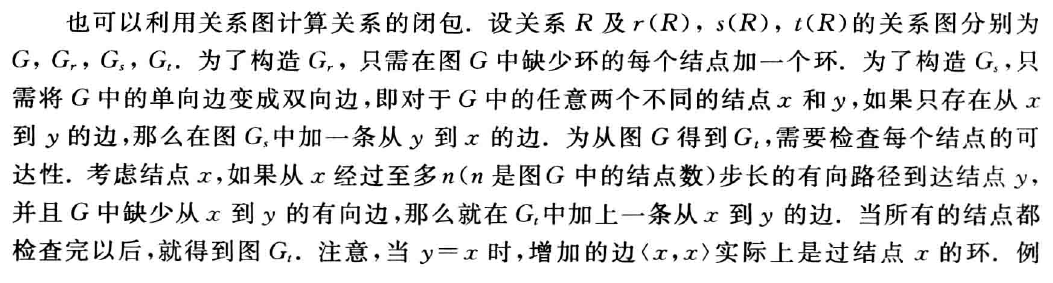#### Warshall算法

🤔 必考必考，行加到列对应1所在行上。

## 等价

### 等价关系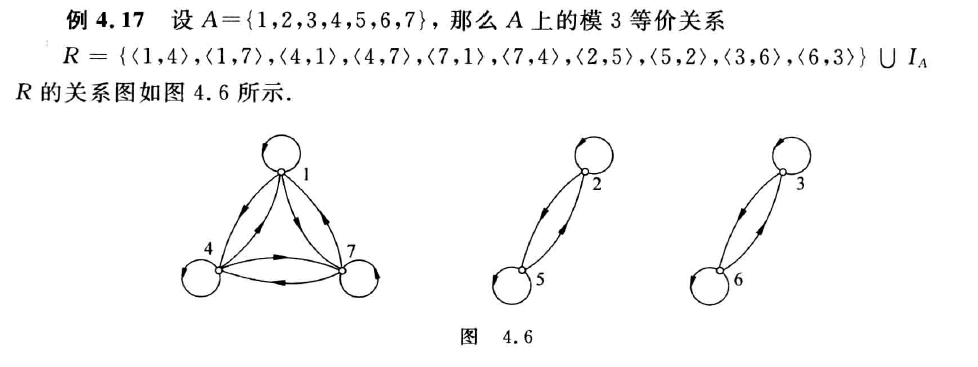### 等价类和商集

#### 定义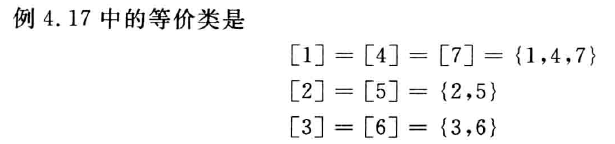#### 性质

• $\forall x\in A,[x]$是$A$的非空子集
• $\forall x,y\in A$，如果$xRy$，则$[x]=[y]$
• $\forall x,y\in A$，如果$xRy$不成立，则$[x]$与$[y]$不交
• $\bigcup_{x\in A}[x]=A$，即$A$中元素构成的所有等价类的并集等于$A$

#### 商集

$A$上的全体等价类构成的集合称作$A$关于等价关系$R$的商集，记作$A/R$，即
$$A/R={[x]_R|x\in A}$$

### 集合的划分

#### 定义

1. $\emptyset\notin\pi$（无空集）
2. $\forall x\forall y(x,y\in\pi\land x\neq y\rightarrow x\cap y=\emptyset)$（元素无公共部分）
3. $\bigcup_{x\in\pi}x=A$（拼起来是完整的）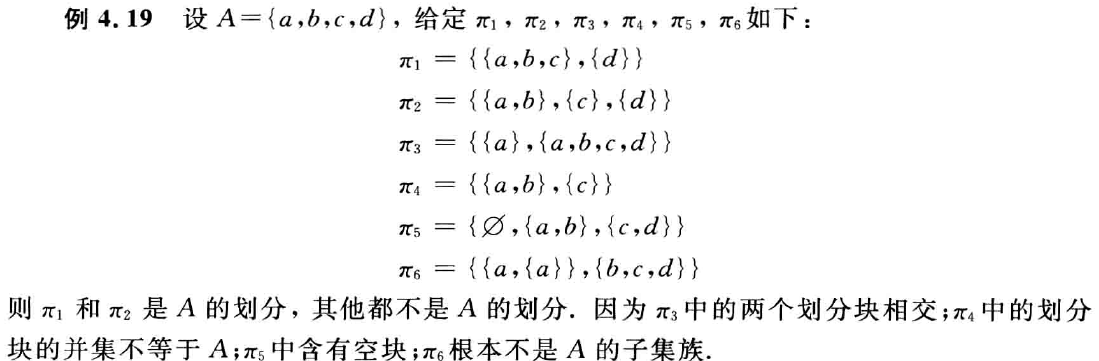• 商集$A/R$就是$A$的一个划分，所以又可以定义商集$R$

$$R={\langle x,y\rangle|x,y\in A\land x与y在\pi 的同一划分块中 }$$

## 偏序

### 偏序关系

#### 拟序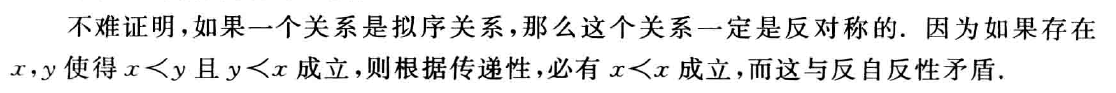#### 覆盖

$T={\langle x,y\rangle|\langle x,y\rangle\in R且y覆盖x}$
$T$的自反传递闭包$rt(T)$就等于$R$

### 偏序集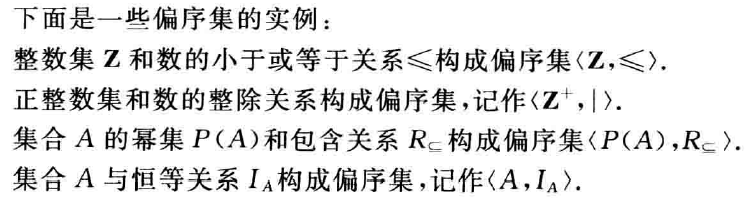#### 哈斯图

• 每个结点没有环
• 两个联通结点之间的序关系通过结点位置的高低表示，位置低的元素顺序在前
• 具有覆盖关系的两个结点之间连边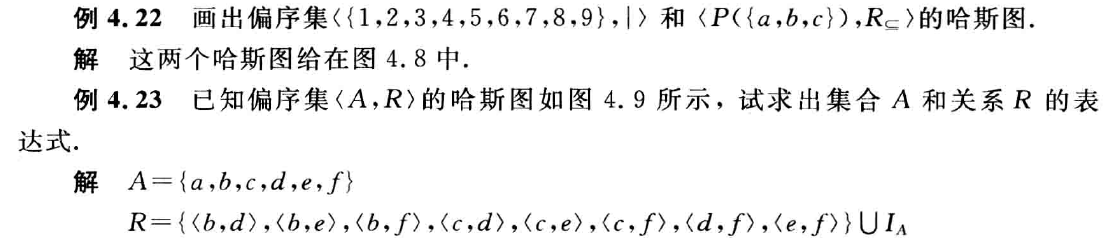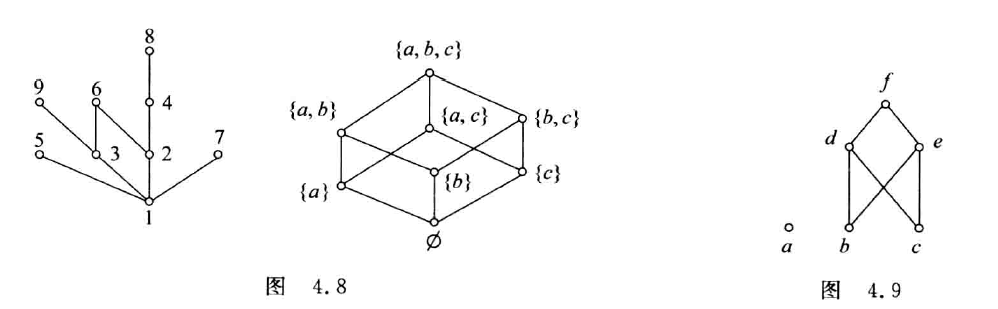#### 特殊元素或子集

• 若$\forall x(x\in B\rightarrow y\preceq x)$成立，则称$y$为$B$的最小元
• 若$\forall x(x\in B\rightarrow x\preceq y)$成立，则称$y$为$B$的最大元
• 若$\forall x(x\in B\land x\preceq y\rightarrow x=y)$成立，则称$y$为$B$的极小元
• 若$\forall x(x\in B\land y\preceq x\rightarrow x=y)$成立，则称$y$为$B$的极大元

• 对于有穷集，极小元和极大元一定存在，还可能存在多个
• 最小元和最大元不一定存在，如果存在一定唯一
• 最小元一定是极小元，最大元一定是极大元
• 孤立结点既是极小元，也是极大元

• 若$\forall x(x\in B\rightarrow x\preceq y)$成立，则称$y$为$B$的上界
• 若$\forall x(x\in B\rightarrow y\preceq x)$成立，则称$y$为$B$的下界
• 令$C={y|y为B的上界}$，则称$C$的最小元为$B$的最小上界上确界
• 令$D={y|y为B的下界}$，则称$D$的最大元为$B$的最大下界下确界

• 上界，下界，最大下界，最小上界不一定存在
• 如果下界，上界存在，也不一定是唯一的
• 最大下界，最小上界如果存在，则是唯一的
• 子集$B$的最小元就是他的最大下界，最大元就是他的最小上界；反之不对

（记住：这里如果是子集，应当将子集当成一个单独的整体，而不受全集的影响。）

#### 特殊子集

• 若$\forall x,y\in B$，$x$与$y$都是可比的，则称$B$是$A$中的一条，$B$中的元素个数称为链的长度
• 若$\forall x,y\in B,x\neq y$，$x$与$y$都是不可比的，则称$B$是$A$中的一条反链，$B$中的元素个数称为反链的长度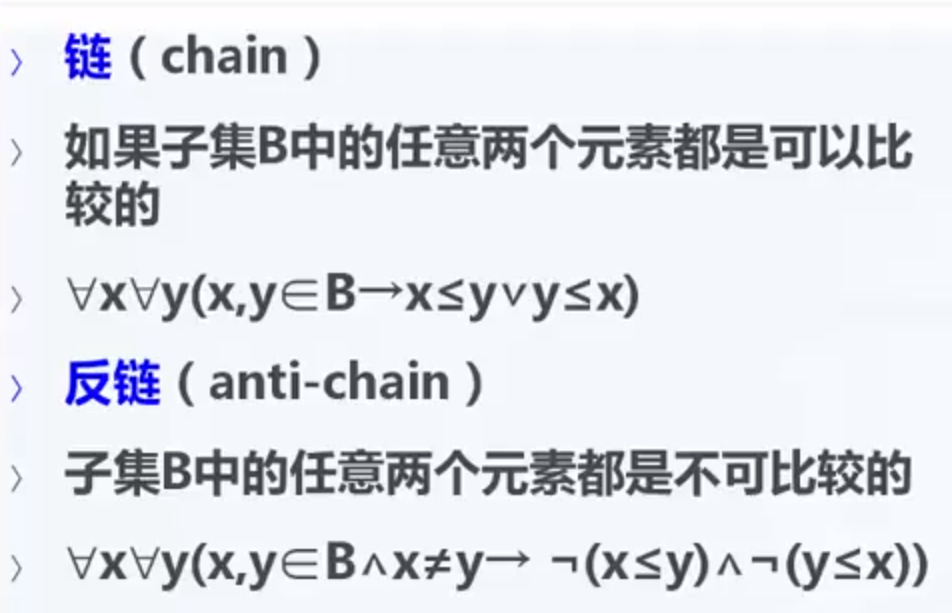• 设$\langle A,\preceq\rangle$为偏序集，若$A$中最长链的长度为$n$，则该偏序集可以分解为$n$条不相交的反链

## 函数

### 基本概念

• $domf=A$
• $ranf\subseteq B$

#### B上A

$$B^A={f|f:A\rightarrow B}$$

#### 重要实例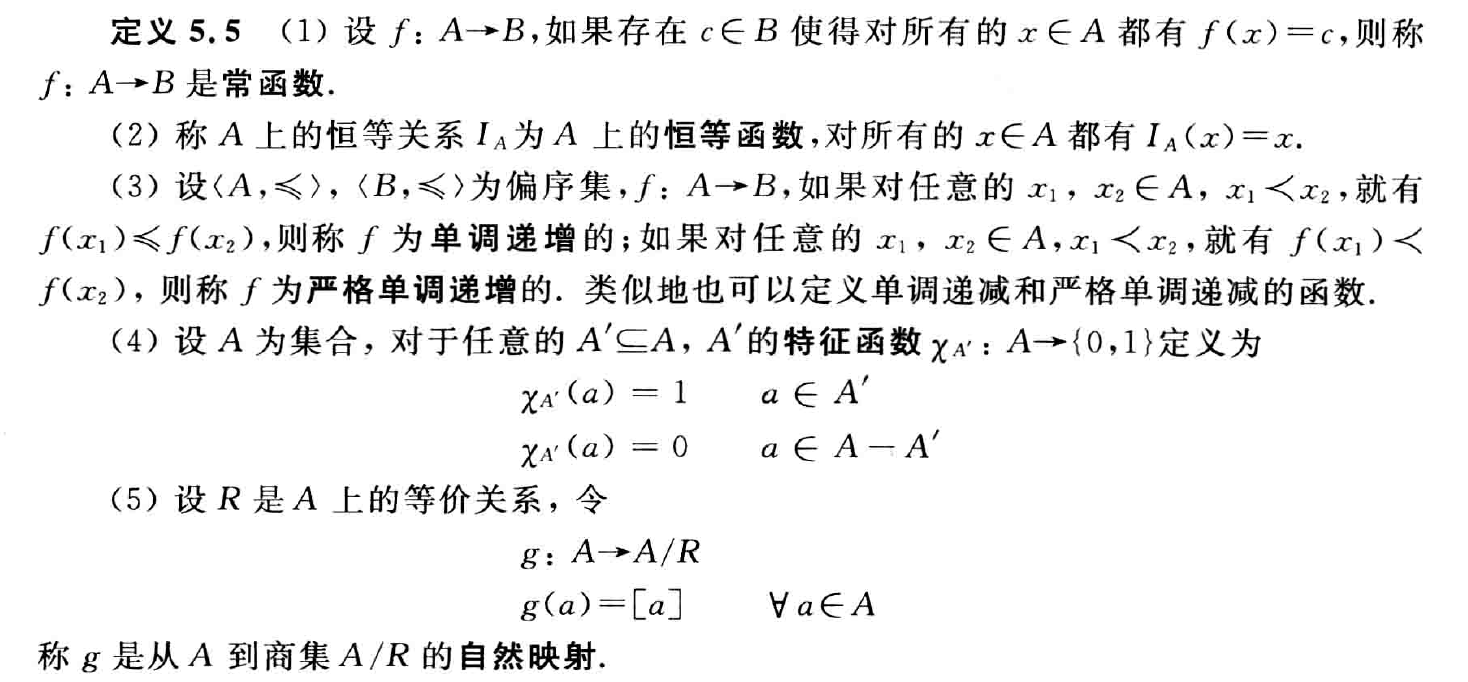#### 像和原像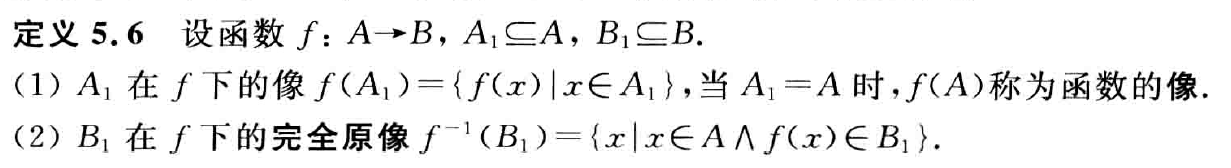### 函数特殊运算

Author:
Reprint policy: All articles in this blog are used except for special statements CC BY 4.0 reprint policy. If reproduced, please indicate source Luminolt !
TOC